# ShymeanV0.7.0

<!--more-->

## 1. 运动

### 1.1. 直线运动

``````位置 = 位置 + 速度

``````
// 初始化
start(){
this.x = 0
this.y = 0
this.dx = 1 // 水平方向的速度
this.dy = 2 // 竖直方向的速度
}

// 每一帧绘制
update(){
this.x += this.dx
this.y += this.dy
}``````

``````update(){
// objectWidth物体宽度，screenWidth窗口宽度，假设都已左上角为坐标原点
// 判断一下是否到达编辑
if(this.x + objectWidth >= screenWidth) {
this.dx = -this.dx
}
// ...
}``````

``````const Input = []

init(){
systemEvent.on(KEY_DOWN, (e)=>{
Input[e.keyCode] = true
})

systemEvent.on(KEY_UP, (e)=>{
Input[e.keyCode] = false
})
}

update(){
if(Input[KEY_CODE.left]) {
// 朝左移动
}
if(Input[KEY_CODE.up]) {
// 朝上移动
}
}``````

### 1.2. 圆周运动

``````let fAngle = 0;

const centerPoint = {
x: 250,
y: 250,
};

update (){
fAngle += (2 * Math.PI) / 360; // 每帧增加的弧度

if (fAngle > 2 * Math.PI) {
fAngle -= 2 * Math.PI; // 避免弧度过大导致精度丢失
}

this.node.x = centerPoint.x + raduis * Math.cos(fAngle);
this.node.y = centerPoint.y + raduis * Math.sin(fAngle);
};``````

``w = 2*PI / T``

## 2. 向量

### 2.1. 加减乘除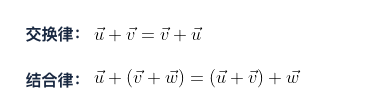``````// 延长3倍
new Vec2(1,1).multiply(3) //  Vec2 { x: 3, y: 3 }
// 缩短1倍
new Vec2(4，2).divide(2) //  Vec2 { x: 2, y: 1 }``````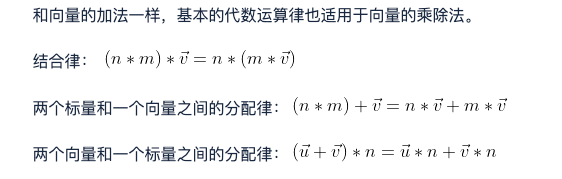### 2.2. 向量的大小与归一化

``new Vec2(3, 4).length(); // 5``

``````N = V(3,4) / |V(3,4)|
N = V(3,4) / 5
N = V(0.6, 0.8)``````

``const dir = new Vec2(3, 4).normalize(); // 与Vec2(3,4) 方向相同但单位为1的向量``

### 2.3. 向量的方向角度

``````cosd = |vy| / |v|
sind = |vx| / |v|``````

### 2.4. 点乘

``u . v = ux * vx + uy * vy````u . v = |u| * |v| * cosd``

``Nu = u / |u|``

``cosa = Nu * Nv``

### 2.5. 叉乘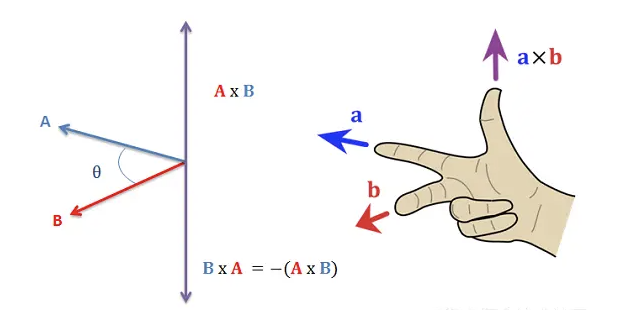## 3. 向量的应用

### 3.1. 获取两个点之间的距离

``````let p1 = new Vec2(1, 2);
let p2 = new Vec2(3, 4);

p1.distance(p2); // 两点之间的距离``````

``p2.subtract(p1).length(); // 与上面结果相同``

### 3.2. 让一个对象面朝另一个对象

``````let p1 = new Vec2(1, 1);
let p2 = new Vec2(2, 0);
let angle = new Vec2(1, 0).angleTo(p2.subtract(p1)); // 与x轴正方向的夹角
let degree = (angle / Math.PI) * 180; // -45``````

### 3.3. 物体直线移动

• 位置向量给我们一个相对于原点的位置
• 速度向量告诉我们如何从一个位置移动到另一个位置

``````let location = new Vec2(4, 4);
let velocity = new Vec2(1, 2);``````

``````function update() {
console.log(location);
}
update(); // Vec2 { x: 5, y: 6 }
update(); // Vec2 { x: 6, y: 8 }
update(); // Vec2 { x: 7, y: 10 }``````

``````let acceleration = new Vec2(-1, 1);

function update() {
velocity.add(acceleration); // vt = v0 + at
console.log(location);
}

update();
update();``````

### 3.4. 圆周运动

``````// 定义圆周运动的一些初始值
const r = 4;
const T = 4;
const w = (2 * Math.PI) / T; // 角速度

let location = new Vec2(r, 0); // 初始位置
function update() {
location.rotate(w); // 每帧旋转的角度
console.log(location);
}

update(); // Vec2 { x: 0, y: 4 }
update(); // Vec2 { x: -4, y: 0 }
update(); // Vec2 { x: -0, y: -4 }
update(); // Vec2 { x: 4, y: -0 }``````

## 4. 三角函数

``弧度 = 2π × (角度 / 360)``

``````角度 = 角度 + 角速度

``````// 让物体顺时针旋转30度
this.node.rotation = +30``````

### 4.1. sohcahtoa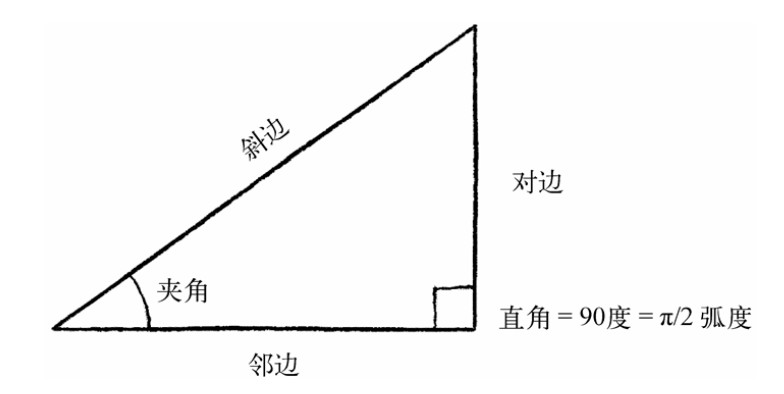• soh：正弦（sin） = 对边(opposite) / 斜边(hypotenuse)
• cah ：余弦（cos） = 邻边(adjacent) / 斜边(hypotenuse)
• toa ：正切（tan） = 对边(hypotenuse) / 邻边(adjacent)

## 5. 力

• 在没有外力作用下孤立质点保持静止或做匀速直线运动（惯性定律）
• 施加于质点的外力等于该质点的质量乘以该质点的加速度（加速度定律）
• 相互作用的两个质点之间的作用力和反作用力总是大小相等，方向相反，作用在同一条直线上(作用力与反作用力定律)

### 5.1. 加速度

``const force = new Vec2(100, 0)``

``````// 这里也可以体会到向量在运算中的威力！！
let acceleration = new Vec2(0, 0) // 物体原本静止
let mass = 1 // 物体的质量
function applyForce(force){
const acc = force.divide(mass) // F = m * a
}

applyForce(new Vec2(100,0))
applyForce(new Vec2(50,0))
// 力的效果会叠加``````

### 5.2. 冲量

``f * dt = m * dv``

## 6. 物理引擎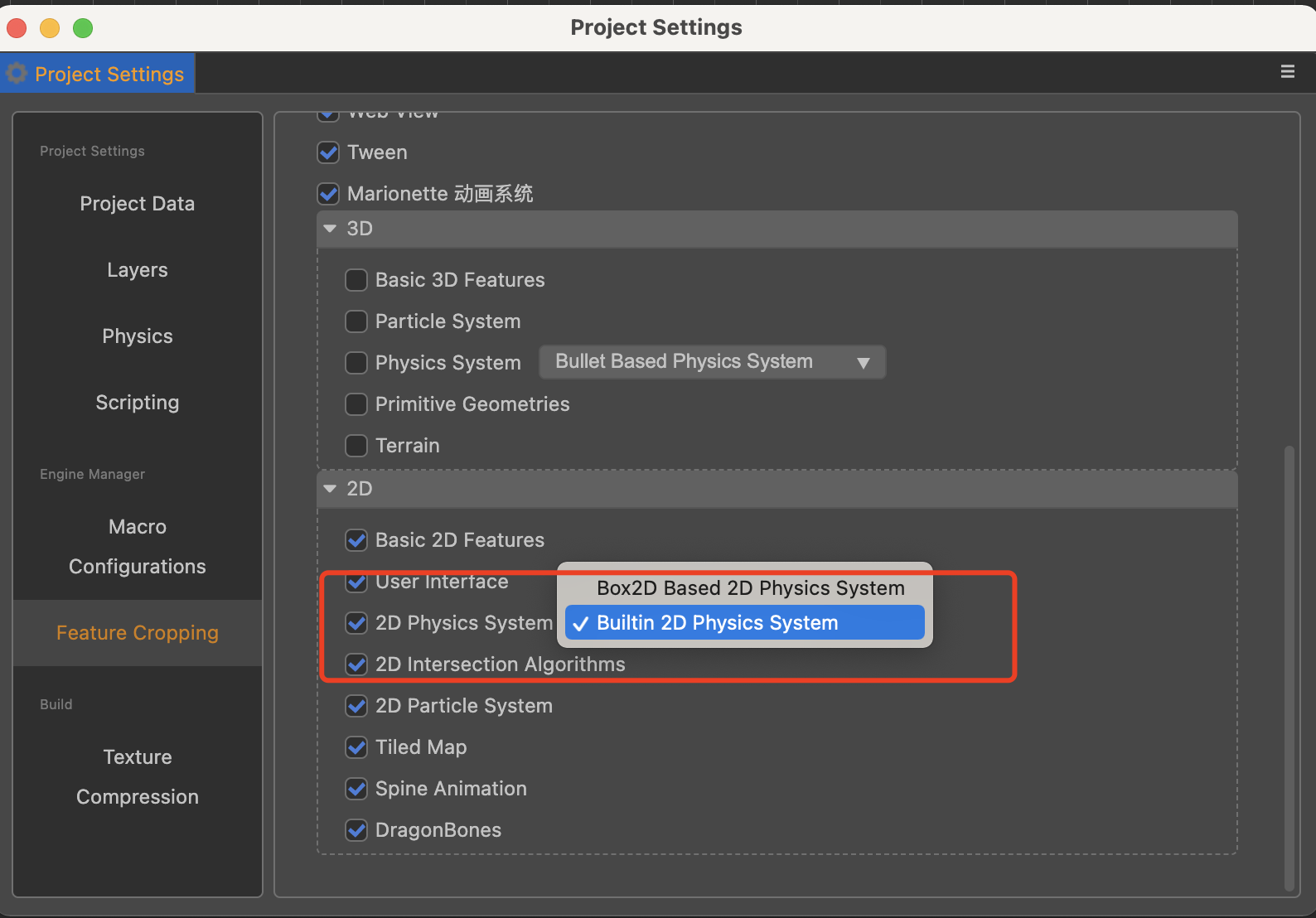• 刚体
• 碰撞体

### 6.1. 刚体

• 动态刚体`Dynamic`，一个“完全模拟”的物体，能执行运动、碰撞，并能感受到环境中的力，在大部分时候我们可能都会使用这种类型
• 静态刚体`Static`，静态物体不能发生位移（速度为0，不会受到力的作用），主要用来模拟某些平台和边界，如地面、墙体等，物理引擎会对静态刚体进行性能优化
• 运动刚体`Kinematic`，0质量，不受力的作用，但是可以设置速度来移动，主要用来设置那些完全由用户控制的对象物体

• 通过重力
• 通过外力
• 通过冲量
• 直接改变速度，`linearVelocity`

### 6.2. 碰撞体

• 如何确定两个物体是否发生碰撞
• 如何确定碰撞后物体各自的速度

• 圆形物体，判断两个圆心是否小于半径之和
• 矩形物体...
• 不规则多边形物体...

### 6.3. ghost collision

 这个问题有个专门的名字，叫做`ghost collision`（鬼打墙）

``````let layer = this.map.getLayer("ground");
let colliderLayer = this.map.getObjectGroup("collider");

const objects = colliderLayer.getObjects();
const object = objects;

const { points, offset } = object;

const ccv2Points = [];
for (let j = 0; j < points.length; j++) {
ccv2Points.push(cc.v2(points[j].x, points[j].y));
}

body.type = cc.RigidBodyType.Static;

collider.offset = cc.v2(offset.x, -offset.y);
collider.points = ccv2Points;
collider.apply();``````

## 7. 自治智能体

• 自治智能体对环境的感知能力是有限的
• 自治智能体需要处理来自外部环境的信息，并由此计算具体的行为
• 自治智能体没有领导者，这一点往往不是特别关心

• 为了模拟怪物的移动，不可能让怪物直接瞬移到枪手的位置
• 怪物知道当前枪手的位置，并及时调整自己的速度方向

• 《游戏人工智能编程案例精粹》
• 《代码本色：用编程模拟自然系统》